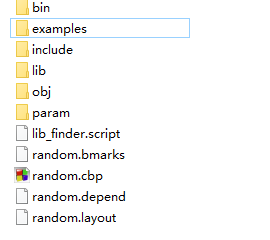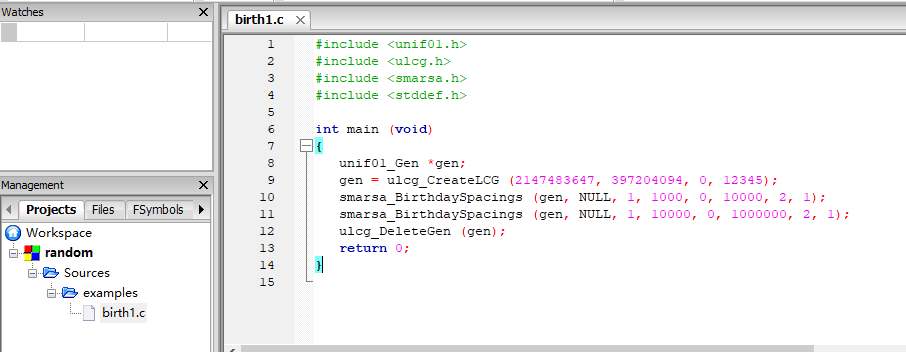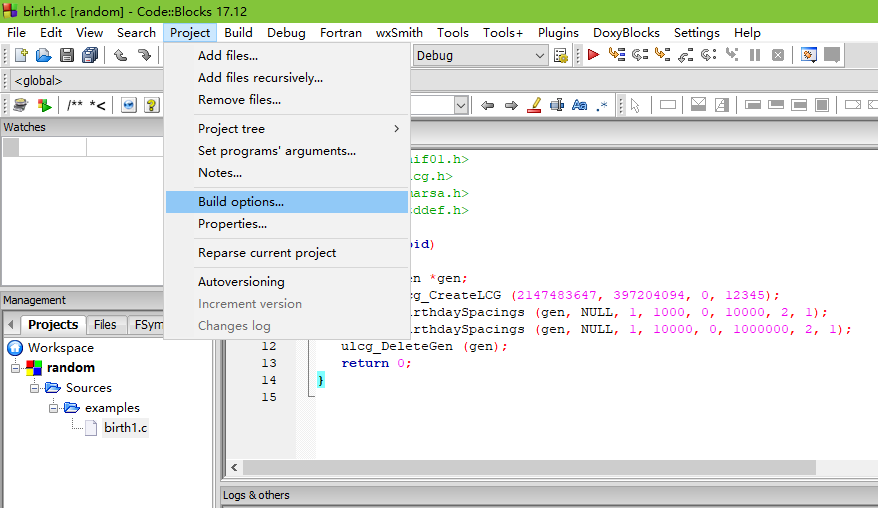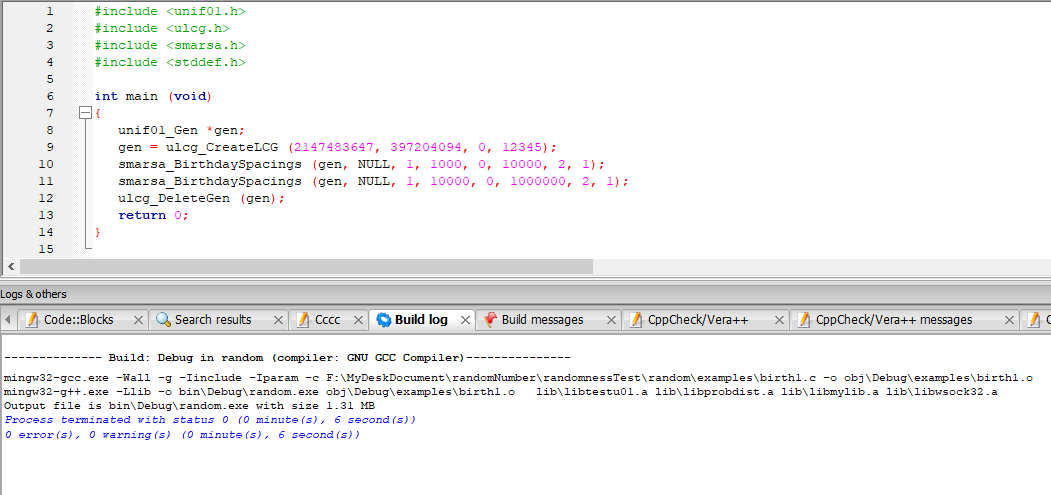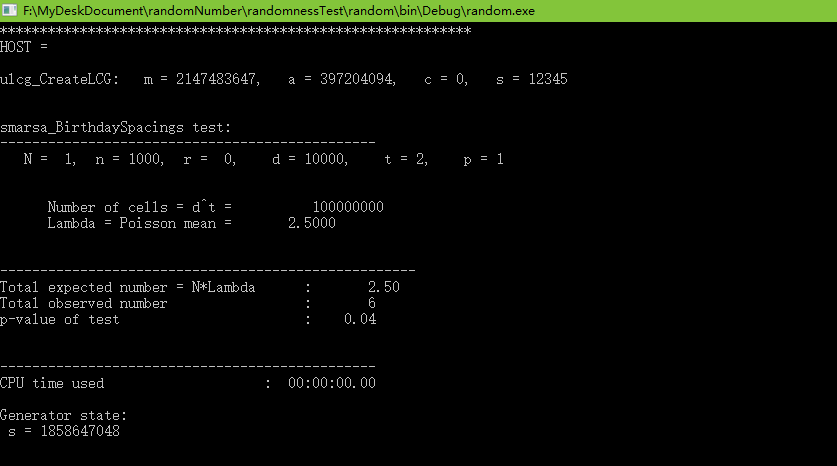# 随机数生成器

• 时间:

"random"（随机）这个词看似简单易懂，但若深究，问题就会上升到量子力学和哲学的高度。

# 一、伪随机数

http://www.math.sci.hiroshima-u.ac.jp/~m-mat/MT/emt.html

### 1.1Mersenne Twister算法的基本过程

Mersenne Twister算法是利用线性反馈移位寄存器（LFSR）产生随机数的，n级的反馈移位寄存器理论上来说最大周期为2^n-1，也就是说理论上可以产生[0,2^n-1]这个范围的随机数。Mersenne Twister算法的n为19937，其周期为梅森素数2^19937-1，这也是该算法名称的由来。

``````算法中用到的变量如下所示：
·w：长度（以bit为单位）
·n：递归长度
·m：周期参数，用作第三阶段的偏移量
·r：低位掩码/低位要提取的位数
·a：旋转矩阵的参数
·f：初始化梅森旋转链所需参数
·b,c：TGFSR的掩码
·s,t：TGFSR的位移量
·u,d,l：额外梅森旋转所需的掩码和位移量

MT19937-32的参数列表如下：
·(w, n, m, r) = (32, 624, 397, 31)
·a = 9908B0DF（16）
·f = 1812433253
·(u, d) = (11, FFFFFFFF16)
·(s, b) = (7, 9D2C568016)
·(t, c) = (15, EFC6000016)
·l = 18``````

### 初始化：（以32位为例）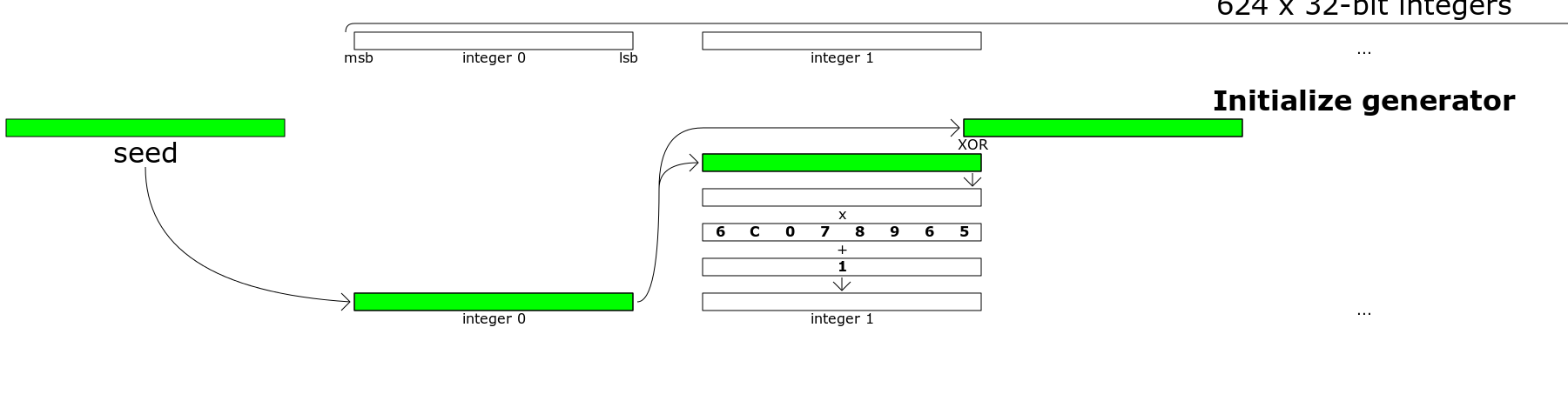MT19937规定了算法1中的f值为1812433253 ，算法将输入的随机种子赋值给第0个状态，剩余的623个状态则用前一状态值的一系列操作进行赋值更新。当执行完这一算法后，全部的624个状态已经填充完毕，之后梅森算法便可以不断地生成伪随机数

### 旋转生成随机数：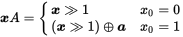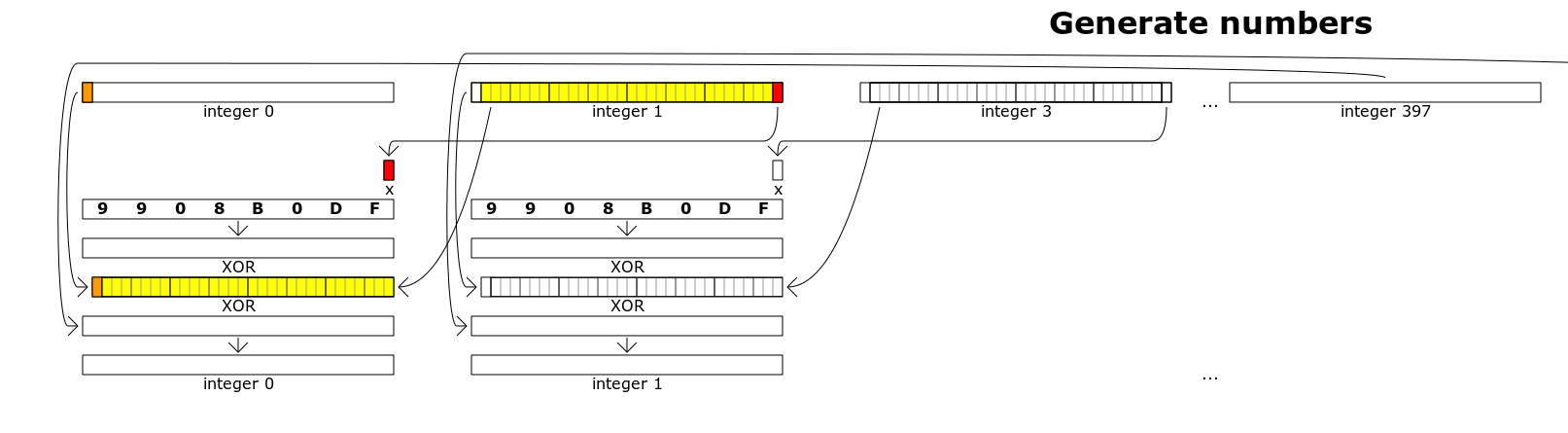### 第三阶段：对于旋转算法所得的结果进行处理

``````b = 0x9d2c5680
c = 0xefc60000
y = mt[i]
y = y XOR (y >> 11)
y = y XOR ((y << 7) AND b)
y = y XOR ((y << 15) AND c)
y = y XOR (y >> 18)``````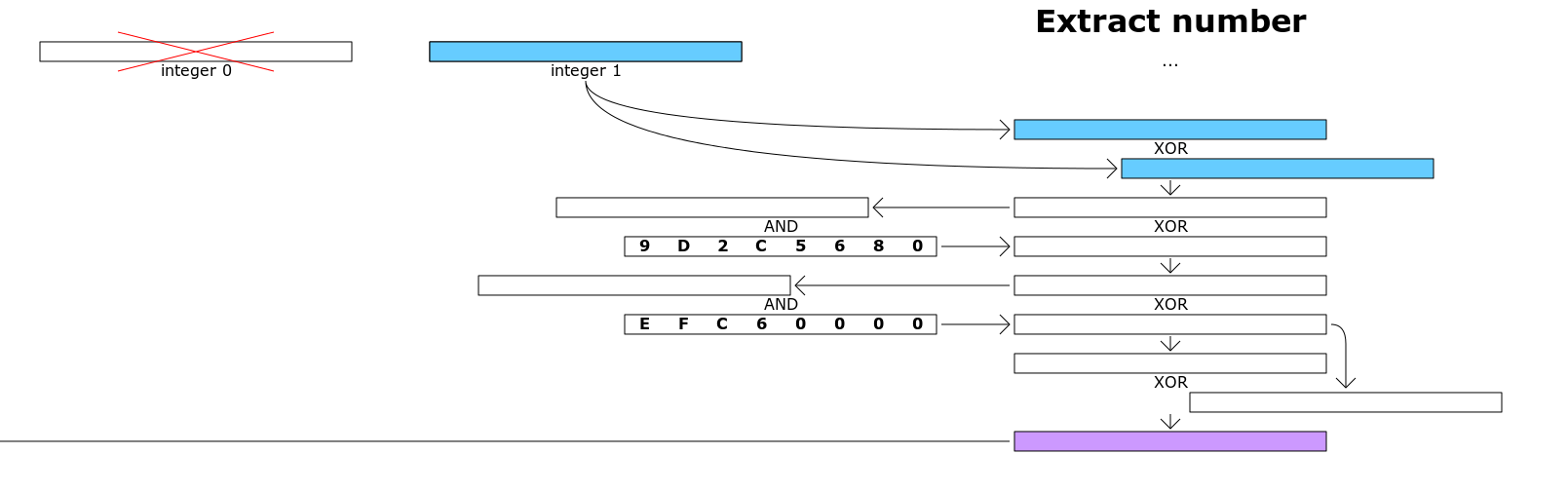### 1.2Mersenne Twister算法的相关定义简介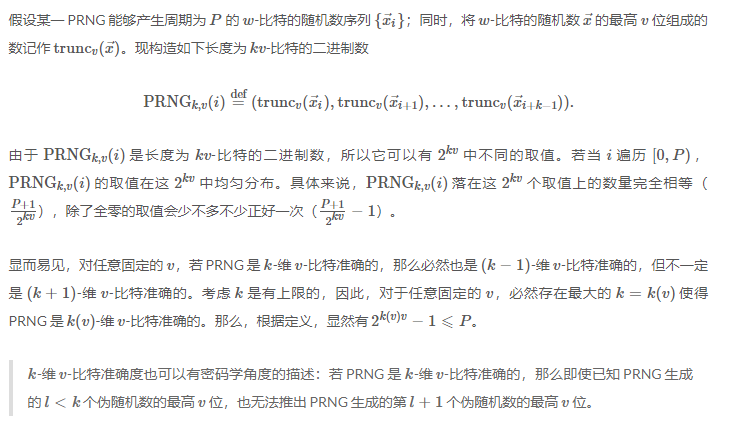以梅森旋转算法为例简单讲解一下：该算法产生的是周期为P=2^19927-1，w=32比特的随机序列，现在假设用该算法产生了P个随机数组成的序列{Xi}，i的取值范围为[0,P).   已经知道该算法是623维32位比特准确的。也就是说对任何k<623,v<32的选取，都是k-维v-比特准确的。   假设k=3，v=2，即取每相邻的三个数（如X0,X1,X2）的最高两个比特位组成一个新的二进制数（如：010011），则这个二进制数的可能的取值有2^(6)=64中可能性，按此方法遍历{Xi}，生成P+1个这样的6比特二进制数，则每种可能的取值在所有P+1个数中所占的比例为1/64.

### 1.3Mersenne Twister算法代码（官网最新修改版本）

``````/*
A C-program for MT19937, with initialization improved 2002/1/26.
Coded by Takuji Nishimura and Makoto Matsumoto.

Before using, initialize the state by using init_genrand(seed)
or init_by_array(init_key, key_length).

Copyright (C) 1997 - 2002, Makoto Matsumoto and Takuji Nishimura,

Redistribution and use in source and binary forms, with or without
modification, are permitted provided that the following conditions
are met:

1. Redistributions of source code must retain the above copyright
notice, this list of conditions and the following disclaimer.

2. Redistributions in binary form must reproduce the above copyright
notice, this list of conditions and the following disclaimer in the
documentation and/or other materials provided with the distribution.

3. The names of its contributors may not be used to endorse or promote
products derived from this software without specific prior written
permission.

THIS SOFTWARE IS PROVIDED BY THE COPYRIGHT HOLDERS AND CONTRIBUTORS
"AS IS" AND ANY EXPRESS OR IMPLIED WARRANTIES, INCLUDING, BUT NOT
LIMITED TO, THE IMPLIED WARRANTIES OF MERCHANTABILITY AND FITNESS FOR
A PARTICULAR PURPOSE ARE DISCLAIMED.  IN NO EVENT SHALL THE COPYRIGHT OWNER OR
CONTRIBUTORS BE LIABLE FOR ANY DIRECT, INDIRECT, INCIDENTAL, SPECIAL,
EXEMPLARY, OR CONSEQUENTIAL DAMAGES (INCLUDING, BUT NOT LIMITED TO,
PROCUREMENT OF SUBSTITUTE GOODS OR SERVICES; LOSS OF USE, DATA, OR
PROFITS; OR BUSINESS INTERRUPTION) HOWEVER CAUSED AND ON ANY THEORY OF
LIABILITY, WHETHER IN CONTRACT, STRICT LIABILITY, OR TORT (INCLUDING
NEGLIGENCE OR OTHERWISE) ARISING IN ANY WAY OUT OF THE USE OF THIS
SOFTWARE, EVEN IF ADVISED OF THE POSSIBILITY OF SUCH DAMAGE.

Any feedback is very welcome.
http://www.math.sci.hiroshima-u.ac.jp/~m-mat/MT/emt.html
email: m-mat @ math.sci.hiroshima-u.ac.jp (remove space)
*/

#include <stdio.h>

/* Period parameters */
#define N 624
#define M 397
#define MATRIX_A 0x9908b0dfUL   /* constant vector a */
#define UPPER_MASK 0x80000000UL /* most significant w-r bits */
#define LOWER_MASK 0x7fffffffUL /* least significant r bits */

static unsigned long mt[N]; /* the array for the state vector  */
static int mti = N + 1; /* mti==N+1 means mt[N] is not initialized */

/* initializes mt[N] with a seed */
void init_genrand(unsigned long s)
{
mt = s & 0xffffffffUL;
for (mti = 1; mti<N; mti++) {
mt[mti] =
(1812433253UL * (mt[mti - 1] ^ (mt[mti - 1] >> 30)) + mti);
/* See Knuth TAOCP Vol2. 3rd Ed. P.106 for multiplier. */
/* In the previous versions, MSBs of the seed affect   */
/* only MSBs of the array mt[].                        */
/* 2002/01/09 modified by Makoto Matsumoto             */
mt[mti] &= 0xffffffffUL;
/* for >32 bit machines */
}
}

/* initialize by an array with array-length */
/* init_key is the array for initializing keys */
/* key_length is its length */
/* slight change for C++, 2004/2/26 */
void init_by_array(unsigned long init_key[], int key_length)
{
int i, j, k;
init_genrand(19650218UL);
i = 1; j = 0;
k = (N>key_length ? N : key_length);
for (; k; k--) {
mt[i] = (mt[i] ^ ((mt[i - 1] ^ (mt[i - 1] >> 30)) * 1664525UL))
+ init_key[j] + j; /* non linear */
mt[i] &= 0xffffffffUL; /* for WORDSIZE > 32 machines */
i++; j++;
if (i >= N) { mt = mt[N - 1]; i = 1; }
if (j >= key_length) j = 0;
}
for (k = N - 1; k; k--) {
mt[i] = (mt[i] ^ ((mt[i - 1] ^ (mt[i - 1] >> 30)) * 1566083941UL))
- i; /* non linear */
mt[i] &= 0xffffffffUL; /* for WORDSIZE > 32 machines */
i++;
if (i >= N) { mt = mt[N - 1]; i = 1; }
}

mt = 0x80000000UL; /* MSB is 1; assuring non-zero initial array */
}

/* generates a random number on [0,0xffffffff]-interval */
unsigned long genrand_int32(void)
{
unsigned long y;
static unsigned long mag01 = { 0x0UL, MATRIX_A };
/* mag01[x] = x * MATRIX_A  for x=0,1 */

if (mti >= N) { /* generate N words at one time */
int kk;

if (mti == N + 1)   /* if init_genrand() has not been called, */
init_genrand(5489UL); /* a default initial seed is used */

for (kk = 0; kk<N - M; kk++) {
mt[kk] = mt[kk + M] ^ (y >> 1) ^ mag01[y & 0x1UL];
}
for (; kk<N - 1; kk++) {
mt[kk] = mt[kk + (M - N)] ^ (y >> 1) ^ mag01[y & 0x1UL];
}
mt[N - 1] = mt[M - 1] ^ (y >> 1) ^ mag01[y & 0x1UL];

mti = 0;
}

y = mt[mti++];

/* Tempering */
y ^= (y >> 11);
y ^= (y << 7) & 0x9d2c5680UL;
y ^= (y << 15) & 0xefc60000UL;
y ^= (y >> 18);

return y;
}

/* generates a random number on [0,0x7fffffff]-interval */
long genrand_int31(void)
{
return (long)(genrand_int32() >> 1);
}

/* generates a random number on [0,1]-real-interval */
double genrand_real1(void)
{
return genrand_int32()*(1.0 / 4294967295.0);
/* divided by 2^32-1 */
}

/* generates a random number on [0,1)-real-interval */
double genrand_real2(void)
{
return genrand_int32()*(1.0 / 4294967296.0);
/* divided by 2^32 */
}

/* generates a random number on (0,1)-real-interval */
double genrand_real3(void)
{
return (((double)genrand_int32()) + 0.5)*(1.0 / 4294967296.0);
/* divided by 2^32 */
}

/* generates a random number on [0,1) with 53-bit resolution*/
double genrand_res53(void)
{
unsigned long a = genrand_int32() >> 5, b = genrand_int32() >> 6;
return(a*67108864.0 + b)*(1.0 / 9007199254740992.0);
}
/* These real versions are due to Isaku Wada, 2002/01/09 added */

int main(void)
{
int i;
unsigned long init = { 0x123, 0x234, 0x345, 0x456 }, length = 4;
init_by_array(init, length);
printf("1000 outputs of genrand_int32()\n");
for (i = 0; i<1000; i++) {
printf("%10lu ", genrand_int32());
if (i % 5 == 4) printf("\n");
}
printf("\n1000 outputs of genrand_real2()\n");
for (i = 0; i<1000; i++) {
printf("%10.8f ", genrand_real2());
if (i % 5 == 4) printf("\n");
}
return 0;
}
``````

MATLAB中有一页主要介绍其使用的各种伪随机数生成算法

MATLAB随机数生成算法介绍

# 二、“真”随机数

1.https://www.random.org

2.http://qrng.anu.edu.au

3.http://www.fourmilab.ch/hotbits/

### 三、随机性测试

The problems with randomness is you can never be sure

A Statistical Test Suite for Random and Pseudorandom Number Generators for Cryptographic Applications

https://en.wikipedia.org/wiki/Diehard_tests

http://simul.iro.umontreal.ca/testu01/tu01.html

https://csrc.nist.gov/Projects/Random-Bit-Generation/Documentation-and-Software

### 3.1 TestU01的安装与使用（windows）lib里面是一些库文件，include里面是各种算法的头文件，share里面有参数文件夹param（各种算法的参数设置），和各种例子examples